# JP3970623B2 - Variable gain amplifier - Google Patents

## Info

Publication number
JP3970623B2
JP3970623B2 JP2002029742A JP2002029742A JP3970623B2 JP 3970623 B2 JP3970623 B2 JP 3970623B2 JP 2002029742 A JP2002029742 A JP 2002029742A JP 2002029742 A JP2002029742 A JP 2002029742A JP 3970623 B2 JP3970623 B2 JP 3970623B2
Authority
JP
Japan
Prior art keywords
transistor
current
circuit
variable gain
connected
Prior art date
Legal status (The legal status is an assumption and is not a legal conclusion. Google has not performed a legal analysis and makes no representation as to the accuracy of the status listed.)
Active
Application number
JP2002029742A
Other languages
Japanese (ja)
Other versions
JP2002330039A (en
Inventor

Original Assignee
シャープ株式会社
Priority date (The priority date is an assumption and is not a legal conclusion. Google has not performed a legal analysis and makes no representation as to the accuracy of the date listed.)
Filing date
Publication date
Priority to JP2001-55482 priority Critical
Priority to JP2001055482 priority
Application filed by シャープ株式会社 filed Critical シャープ株式会社
Priority to JP2002029742A priority patent/JP3970623B2/en
Publication of JP2002330039A publication Critical patent/JP2002330039A/en
Application granted granted Critical
Publication of JP3970623B2 publication Critical patent/JP3970623B2/en
Application status is Active legal-status Critical
Anticipated expiration legal-status Critical

## Images

•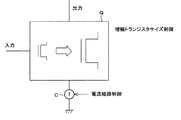•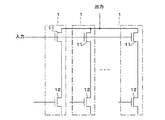•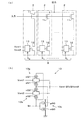•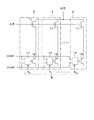•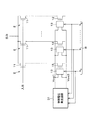•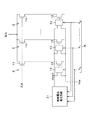•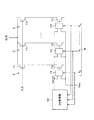•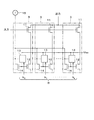•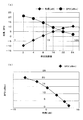•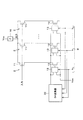•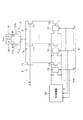•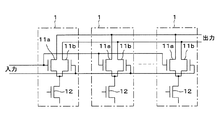•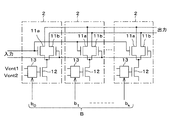•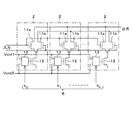••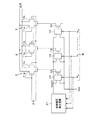••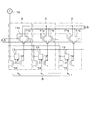•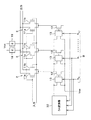•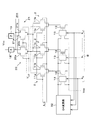•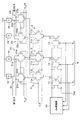•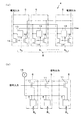•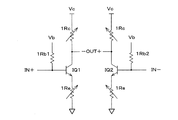••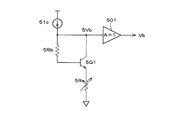•## Classifications

• HELECTRICITY
• H03BASIC ELECTRONIC CIRCUITRY
• H03FAMPLIFIERS
• H03F3/00Amplifiers with only discharge tubes or only semiconductor devices as amplifying elements
• H03F3/45Differential amplifiers
• H03F3/45071Differential amplifiers with semiconductor devices only
• H03F3/45076Differential amplifiers with semiconductor devices only characterised by the way of implementation of the active amplifying circuit in the differential amplifier
• H03F3/45179Differential amplifiers with semiconductor devices only characterised by the way of implementation of the active amplifying circuit in the differential amplifier using MOSFET transistors as the active amplifying circuit
• H03F3/45183Long tailed pairs
• HELECTRICITY
• H03BASIC ELECTRONIC CIRCUITRY
• H03GCONTROL OF AMPLIFICATION
• H03G3/00Gain control in amplifiers or frequency changers without distortion of the input signal
• H03G3/20Automatic control
• H03G3/30Automatic control in amplifiers having semiconductor devices
• H03G3/3036Automatic control in amplifiers having semiconductor devices in high-frequency amplifiers or in frequency-changers
• H03G3/3042Automatic control in amplifiers having semiconductor devices in high-frequency amplifiers or in frequency-changers in modulators, frequency-changers, transmitters or power amplifiers

## Description


BACKGROUND OF THE INVENTION
The present invention relates to a variable gain amplifier having a high linearity constituted by using a semiconductor element, and more particularly to a variable gain amplifier effective for realizing a broadband wireless communication device.

[Prior art]
Wideband wireless communication devices such as cable and radio wave tuners for TV broadcasting use variable gain amplifiers with high linearity (low distortion) to suppress inter-channel intermodulation interference when strong signals are input. Need. In a tuner configured using conventional discrete components, a signal attenuator is configured using highly linear PIN diode components, and a strong signal is not input to the amplifier to generate an intermodulation interference signal. I have suppressed it. However, a PIN diode cannot be formed on a commonly used semiconductor integrated circuit such as CMOS or Bipolar. For this reason, in order to configure a wireless communication device with a semiconductor integrated circuit, a method for realizing different variable gain amplifiers is required.

A configuration method of such a variable gain amplifier is disclosed in US Pat. No. 6,100,761 (Highly Linear Variable-Gain Low Noise Amplifier). As shown in FIG. 23, this amplifier includes an NPN transconductor pair including transistors IQ1 and IQ2. The power supply voltage Vc is applied to the collectors of the transistors IQ1 and IQ2 via the variable resistor 1Rc, and the emitter is connected to the GND line via the variable resistor 1Re. The bases of the transistors IQ1 and IQ2 receive inputs IN + and IN-, respectively, and a bias voltage Vb via the resistors 1Rb1 and 1Rb2, respectively.

The gain of this amplifier is 1Rc / (1 / gm + 1Re). Here, gm represents the transconductance of the transistors IQ1 and IQ2. In the above amplifier, as shown in FIG. 24, 1Re is constituted by a resistor ladder composed of resistors Rn1, Rn2, Rn3, and nMOS switches Mn1, Mn2, Mn3,. Realized. The nMOS switches Mn1, Mn2, Mn3,... are controlled to be turned on and off by the voltages Vn1, Vn2, Vn3,.

[Problems to be solved by the invention]
However, the above amplifier has the following three problems.

First, in order to design the resistance ladder with high accuracy, it is necessary to keep the on-resistance of the nMOS switches Mn1, Mn2, Mn3. However, for example, in order to make a switch having an on-resistance of 1Ω using a standard 0.25 μm CMOS process, a huge transistor having a channel width of 1000 μm is required. Therefore, in order to realize the above-described resistance ladder, a plurality of such huge transistors are required, which increases the mounting area.

Second, when the resistance value of the variable resistor 1Re is changed, the current flowing through the circuit changes, and the operating point of the transistor fluctuates. In order to suppress this variation, a bias voltage Vb adjusting circuit as shown in FIG. 25 is provided. In this adjustment circuit, a resistor 5Rb is connected between the collector and base of the transistor 5Q1, and a variable resistor 5Re is provided between the emitter of the transistor 5Q1 and the GND line. In addition, a bias current 51c flows through the connection point between the resistor 5Rb and the collector, and the voltage 5Vb appearing at the collector is output as the bias voltage Vb through the buffer amplifier 501. However, since this adjustment circuit includes the resistor 5Rb serving as a resistor ladder replica, the mounting area increases.

Third, although the bipolar transistor is used in the configuration shown in FIG. 23 and FIG. 25, the bipolar transistor is replaced with a MOS transistor in order to realize a similar circuit using a CMOS process cheaper than the BiCMOS process. Think about things. Here, the current I flowing through the MOS transistor is
I = K · (W / L) · Vod2                                 ... given by (1).

Here, K is a constant determined by the process, W is the channel width of the transistor, and L is the channel length of the transistor. Further, Vod = Vgs−Vth and Vgs = Vg−Vs. Here, Vg is a gate voltage, Vs is a source voltage, and Vth is a threshold voltage of the transistor. Then, even if the resistance value of the variable resistor 1Re is changed in order to reduce the gain, the adjustment circuit for the bias voltage Vb works to pass a constant current, and the voltage Vod does not change.

By the way, IIP3 (third-order input intercept point) which is an index representing the third-order distortion component of the circuit and is an input value in which the third-order intermodulation component when the two-tone input is given becomes the same value as the first-order component ), "RF Linearity of Short-Channel MOSFETs, Theerachet Soorapanth and Thomas H. Lee, First International Workshop on Design of Mixed-Mode Integrated Circuits and Applications, Cancun, Mexico, July, 28-30, 1997, pp.81 -84 ”. According to FIG. 5 of this document, IIP3 of the MOS transistor is determined by the value of Vod. Therefore, even if the gain of the amplifier is changed by changing the resistance value of the variable resistor 1Re, IIP3 does not change.

The present invention has been made in view of the above circumstances, and an object of the present invention is to realize a variable gain amplifier having a small mounting area that can cause a decrease in gain as an increase in IIP3.

[Means for Solving the Problems]
In order to solve the above problems, the variable gain amplifier of the present invention providesIncluding an amplification transistor for amplifying an input signal and a current control transistor for controlling a current flowing through the amplification transistor, and having a plurality of unit circuits arranged in parallel, the signal inputs of each unit circuit are connected to each other, The signal outputs of the unit circuits are connected to each other, and each unit circuit has a switching control circuit that switches the operation control voltage of the current control transistor to a plurality of values. A voltage generation circuit for generating the operation control voltage based on a switching control signal for switching output, and the operation common to the switching control circuit of each unit circuit A control voltage is input, and the operation control voltage is two voltages, a value that cuts off a current flowing through each unit circuit, and a value that flows a current through each unit circuit. The other operation control voltage is changed according to the number of unit circuits in which the current is cut off, and the total amount of current flowing through the remaining unit circuits is controlled.It is characterized by that.

In the above configuration, when the size (W / L) of the amplification transistor is reduced or increased in a state where the current is kept constant by the current path control unit, the linearity index IIP3 is increased or decreased, and the gain is increased. Decrease or increase. As a result, it is possible to eliminate the inconvenience that the IIP3 does not increase even when the gain is reduced in the variable gain amplifier having the CMOS configuration. In addition, the above-described resistance ladder that has been conventionally used for variable gain is not required, and therefore a large switch for reducing the on-resistance of the switch in the resistance ladder is also unnecessary.

The variable gain amplifier, which is the basis of the present invention, includes a current control transistor that controls the current flowing through the amplification transistor in the current path control unit, and includes the amplification transistor and the current control transistor, The unit circuit further includes a plurality of arranged unit circuits, the signal inputs of the unit circuits are connected to each other, and the signal outputs of the unit circuits are connected to each other.The

In this configuration, the current flowing through the amplification transistor can be independently controlled by the control of the current control transistor. For example, when the current flowing through the amplification transistor of a certain unit circuit is cut off, the amplification transistor of this unit circuit does not contribute to the amplification of the input signal.

In this variable gain amplifier, each unit circuit has a switching control circuit that switches the operation control voltage of the current control transistor to a plurality of values.TheWith this configuration, the size of the amplification transistor and the current flowing through the amplification transistor in the unit circuit can be controlled.

In this variable gain amplifier, the common operation control voltage is input to the switching control circuit of each unit circuit.TheWith this configuration, the number of operation control voltages to be used is reduced.

Also,The operation control voltage is two voltages, a value that cuts off a current flowing through each unit circuit and a value that flows a current through each unit circuit.TheIn such a configuration, when the size of the amplification transistor is reduced by cutting off the current, the amount of flowing current can be kept constant by controlling the operation control voltage so as to be away from the voltage that cuts off the current. .

The variable gain amplifier includes a voltage generation circuit that generates the operation control voltage based on a switching control signal for switching the output of the switching control circuit for each unit circuit.TheWith this configuration, it is possible to change the value of the operation control voltage in accordance with the value of the switching control signal, change the size of the amplification transistor, and control the amount of current flowing through the amplification transistor.

In this variable gain amplifier value, the voltage generation circuit changes the other operation control voltage according to the number of unit circuits in which the current is cut off, and controls the total amount of current flowing in the remaining unit circuits.TheWith this configuration, the current flowing through each unit circuit can be controlled by the entire variable gain amplifier.

From the above, it is possible to realize a variable gain amplifier with a small mounting area that can cause a decrease in gain as an increase in IIP3.

In this variable gain amplifier, it is preferable that the voltage generation circuit generates the operation control voltage so as to control the total current amount to be constant. With this configuration, in a variable gain amplifier including a unit circuit through which a current flows, the gain decrease can be reliably caused to act as an increase in IIP3.

In this variable gain amplifier, the current path control unit includes a current control transistor that controls a current flowing through the amplification transistor, and forms a current mirror together with the amplification transistor, the current control transistor, and the current control transistor. An auxiliary current control transistor, and a switching control circuit that switches the current control transistor and the operation control voltage of the auxiliary current control transistor to a plurality of values. It is preferable to include a plurality of unit circuits to which signal inputs are connected to each other and signal outputs of each unit circuit are connected to each other, and a current source for supplying a constant current to the auxiliary current control transistor.

As a result, a constant current from the current source flows to the amplification transistor by the current control transistor and the auxiliary current control transistor constituting the current mirror. Therefore, a circuit that allows a constant current to flow regardless of the size of the amplification transistor can be realized by simply configuring the current control transistor and the auxiliary current control transistor with MOS transistors. Therefore, the cost of the variable gain amplifier can be reduced.

In each of the variable gain amplifiers including the unit circuit (except for the variable gain amplifier including a current source that supplies a constant current to the auxiliary current control transistor), the current control transistor in each unit circuit is constant. It is preferable to provide a current source for supplying current. With this configuration, the current flowing through the amplifying transistor becomes the current value of the current source, regardless of whether the current control transistor is cut off or conducted (except when all are cut off). Therefore, if the current value of the current source is kept constant and the number of unit circuits to be cut off is increased, IIP3 can be increased when the size of the amplification transistor is reduced and the gain is reduced.

In each of the variable gain amplifiers including the unit circuit, it is preferable that the amplification transistor is an amplification transistor differential pair. With this configuration, a signal obtained from the transistor differential pair becomes a fully differential signal, and a circuit that is highly resistant to fluctuations in the power supply voltage and the ground voltage can be configured.

In each of the variable gain amplifiers including the unit circuit, it is preferable that the output of each unit circuit is connected to a power source via a load impedance. With this configuration, the output current is converted into a voltage, and a voltage input / voltage output type variable gain amplifier can be realized.

Each of the variable gain amplifiers including a unit circuit includes a transistor differential pair in which an output of each unit circuit is connected to a source, and a load impedance that connects each output of the transistor differential pair to a power source. It is preferable that oscillation signals whose phases are shifted by 180 ° are input to the gates of the transistors constituting the transistor differential pair. With this configuration, a mixer circuit having a variable gain control function can be realized. As a result, normally, the amplifier and the mixer circuit to be cascade-connected are combined.

In this variable gain amplifier, a first transistor differential pair in which one of the differential outputs of each amplification transistor differential pair is connected to the source, and a second transistor differential pair in which the other of the differential outputs is connected to the source. A differential pair of first and second transistors whose differential outputs are cross-connected to each other, and a load impedance for connecting each output of the first and second transistor differential pairs to a power source, It is preferable that oscillation signals whose phases are shifted by 180 ° are input to the gates of the transistors constituting the first and second transistor differential pairs. With this configuration, a mixer circuit having a fully differential variable gain control function can be realized. As a result, normally, the amplifier and the mixer circuit to be cascade-connected are combined.

Alternatively, in the variable gain amplifier including the amplification transistor differential pair, the first transistor differential pair in which one of the differential outputs of each amplification transistor differential pair is connected to the source and the other of the differential outputs is A second transistor differential pair connected to the source, the first and second transistor differential pairs having their differential outputs cross-connected, and one of the differential outputs of each amplification transistor differential pair is the source A third transistor differential pair connected to the second transistor and a fourth transistor differential pair in which the other of the differential outputs is connected to the source, and the third and fourth transistor differences whose differential outputs are cross-connected to each other And a load impedance for connecting each output of the first to four-transistor differential pairs to a power source, and about 180 ° between the differential inputs of the first and second transistor differential pairs. Of while shifted first oscillating signal is inputted, it is preferable that the second oscillation signal the first oscillation signal to the differential inputs of the third and fourth differential transistor pair is phase shifted by 90 ° is input.

With this configuration, a signal component is extracted from the outputs of the first and second transistor differential pairs, and a signal component obtained by shifting the signal component by 90 ° is output from the third and fourth transistor differential pairs. Taken from. Thereby, a mixer circuit having a variable gain control function for extracting two different signal components can be realized. As a result, normally, the amplifier and the mixer circuit to be cascade-connected are combined.

In each of the variable gain amplifiers including the unit circuit, the first transistor, the second transistor paired with the first transistor to form a current mirror, and the operation control of the first and second transistors. A plurality of unit current mirror circuits arranged in parallel, the current inputs of each unit current mirror circuit are connected to each other, and the current outputs of each current mirror circuit are connected to each other. Is preferably provided instead of the current path control unit.

Thereby, when the size of the amplification transistor is small, the variable impedance current mirror circuit increases the size of the current control transistor, so that a sufficient current can flow. Therefore, the current copied from the auxiliary current control transistor to the current control transistor does not decrease. Therefore, the current control transistor can be prevented from entering the linear region operation, and the operation region of the current control transistor can be expanded.

DETAILED DESCRIPTION OF THE INVENTION
Each embodiment of the present invention will be described below with reference to FIGS. 1 to 22 and FIG.

First, the basic principle of the variable gain amplifier according to this embodiment will be described.

As shown in FIG. 1, an amplifying transistor unit Q and a current path control circuit C are provided. The current path control circuit C as a current path control unit controls the size of the transistors constituting the amplification transistor unit Q, the IIP3, and the gain by controlling the path of the current flowing through the amplification transistor unit Q.

From the equation (1), the voltage Vod that determines the IIP3 of the MOS transistor is
Vod = {I / (K · W / L)}1/2                           ... represented as (2). On the other hand, the transconductance gm that determines the gain of the MOS transistor is obtained by differentiating the equation (1).
gm = 2 · K · (W / L) · Vod = 2 · {I · K · W / L}1/2 ... (3)

From Equations (2) and (3), when the transistor size W / L decreases or increases while the current I is kept constant by the current control circuit C, the voltage Vod or IIP3 increases or decreases, and the transformer It can be seen that the conductance gm or gain decreases or increases. Based on this phenomenon, the variable gain amplifier of the present invention keeps the current I flowing through the amplification transistor and reduces or increases the size W / L of the transistor, thereby increasing or decreasing IIP3 and decreasing or increasing the gain. Can be made.

The specific configuration of the variable gain amplifier will be described below in each embodiment. In addition, about the component which is common in each embodiment, the same code | symbol is attached.

[Embodiment 1]
As shown in FIG. 2, the variable gain amplifier according to the present embodiment includes a plurality of unit circuits 1 each including a signal input transistor 11 (amplification transistor) and a current control transistor 12. In this unit circuit 1, the source of the signal input transistor 11 and the drain of the current control transistor 12 are connected, and the drain of the signal input transistor 11 is used as a signal output. Unit circuits 1 are arranged in parallel, and signal inputs (gates of the signal input transistors 12) of the unit circuits 1 are connected to each other, and signal outputs are connected to each other, so that a variable transformer having a plurality of control inputs is provided. A conductance (voltage current gain) circuit is configured.

In the variable transconductance circuit configured as described above, by controlling the gate voltage of the current control transistor 12, the current flowing through each unit circuit 1 (signal input transistor 11) can be controlled independently. By applying one power supply voltage (low potential side power supply voltage) to the gate of the current control transistor 12, for example, a ground voltage for an nMOS transistor and a power supply voltage (high potential side power supply voltage) for a pMOS transistor, The current of the unit circuit 1 can be cut off. That is, since the signal input transistor 11 of the unit circuit 1 does not contribute to amplification of the input signal, the substantial size of the signal input transistor 11 can be controlled for each unit circuit 1. Of course, as will be described later, the size of the signal input transistor 11 may be different in each unit circuit 1.

In the above configuration, by making the gate length L of the transistors of each unit circuit 1 common, the size W / L of the signal input transistor 11 can be controlled.

[Embodiment 2]
As shown in FIG. 3A, the variable gain amplifier according to the present embodiment includes a plurality of unit circuits 2 each having a current control changeover switch 13 in addition to the unit circuit 1 in the variable gain amplifier of FIG. The current control changeover switch 13 serving as a changeover control circuit switches and supplies different current control voltages Vcnt1 and Vcnt2 with a changeover control signal B as gate voltages (operation control voltages) to be applied to the gate (input unit) of the current control transistor 12. . The switching control signal B is a digital signal composed of b0, b1,..., Bk (k is an integer of 0 or more) constituting each bit, and the value of each bit is input to each unit circuit 2.

Normally, the switching control signal B is generated according to the magnitude of the input signal or the output signal so that the magnitude of the output signal is constant.

3A shows the case where two current control voltages Vcnt1 and Vcnt2 are switched, three or more current control voltages may be switched.

By such logic control, the size of the signal input transistor 11 contributing to current amplification and the current flowing through the unit circuit 2 can be controlled, and more accurate control can be performed. For example, when B = 0, that is, when (b0, b1,..., Bk) are all "0", all transistors are cut off, so that no output current flows. If any one of (b0, b1,..., Bk) is “1”, the current control transistor 12 in the unit circuit 2 to which it is input operates. Further, when (b0, b1,..., Bk) are all “1”, the current flowing through the current control transistor 12 is maximized.

Specifically, the current control changeover switch 13 includes, for example, transmission gates 13a and 13b and an inverter 13c as shown in FIG. 3B. The transmission gate 13a is formed by connecting a pMOS transistor pQ1 and an nMOS transistor nQ1 in parallel, and the transmission gate 13b is formed by connecting a pMOS transistor pQ2 and an nMOS transistor nQ2 in parallel. The inverter 13c is formed by connecting a pMOS transistor pQ3 and an nMOS transistor nQ3 in series.

Each bit bn (n = 0, 1,..., K) of the switching control signal B is input to the gates of the pMOS transistors pQ2 and pQ3 and the nMOS transistors nQ1 and nQ3. The connection point between the drains of the pMOS transistor pQ3 and the nMOS transistor nQ3 is connected to the gates of the pMOS transistor pQ1 and the nMOS transistor nQ2.

In the current control changeover switch 13 configured as described above, the value of the bit bn is directly input to the gates of the nMOS transistor nQ1 and the pMOS transistor pQ2, while being inverted via the inverter 13c, the pMOS transistor pQ1 and the nMOS Input to the gate of transistor nQ2. As a result, either one of the transmission gates 13a and 13b is opened according to the binary value of the bit bn, and one of the current control voltages Vcnt1 and Vcnt2 is output.

Here, in the configuration of FIG. 3A, each unit circuit 2 has a current control voltage Vcnt1 ·· so that the control of the size of the signal input transistor 11 and the current flowing through the unit circuit 2 is different for each unit circuit 2. Vcnt2 may be set in any way.

Further, as shown in FIG. 4, the current control voltages Vcnt1 and Vcnt2 may be shared by the unit circuits 2. In such a configuration, since only two current control voltages Vcnt1 and Vcnt2 are used, it is not necessary to prepare many voltages, and the amount of current flowing through the signal input transistor 11 can be easily controlled.

For example, the configuration shown in FIG. 5 includes a control voltage generation circuit 31 that changes the current control voltages Vcnt1 and Vcnt2. The control voltage generation circuit 31 controls the values of the current control voltages Vcnt1 and Vcnt2 according to the value of each bit bn of the switching control signal B. As a result, the values of the current control voltages Vcnt1 and Vcnt2 are changed in accordance with the value of each bit bn, thereby changing the size of the signal input transistor 11 contributing to amplification and controlling the amount of current flowing through the signal input transistor. can do.

In the configuration shown in FIG. 6, as a special embodiment of FIG. 5, the current control voltage Vcnt2 (which may be Vcnt1) is one power supply voltage Vss (for blocking the current flowing through the signal input transistor 11). It is fixed at the low potential side (for example, ground voltage).

In such a configuration, when the size of the signal input transistor 11 is reduced by cutting off the current, the current control voltage Vcnt1 (or Vcnt2) is cut off while keeping the amount of current flowing constant. What is necessary is just to control so that it may keep away from the power supply voltage Vss. Further, the control voltage generation circuit 31 changes the other current control voltage Vcnt1 (or Vcnt2) according to the number of unit circuits 2 in which the current is cut off, and the total amount of current flowing through the remaining unit circuits 2 becomes constant. So that it is controlled.

The configuration shown in FIG. 7 is an example for realizing the configuration of FIG. 6, and includes a DA converter 32 as the control voltage generation circuit 31. The DA converter 32 converts the input digital switching control signal B into analog to generate a current control voltage Vcnt1.

[Embodiment 3]
As shown in FIG. 8, the variable gain amplifier according to the present embodiment is configured to simply realize the function of the variable gain amplifier shown in FIGS. 4 to 7 with a MOS transistor. Specifically, this variable gain amplifier includes a plurality of unit circuits 3 in which each unit circuit 2 is further added with a current control transistor 14 as an auxiliary current control transistor, instead of the unit circuit 2 described above, and a current source 15. It has.

The plurality of unit circuits 3 are arranged in parallel as in the unit circuits 2. The signal inputs of the unit circuits 3 are connected to each other, and the signal outputs of the unit circuits 3 are connected to each other.

The source of the signal input transistor 11 and the drain of the current control transistor 12 are connected, and the gate of the current control transistor 12 is connected to the gate of the current control transistor 14 and the output terminal of the current control changeover switch 13. The power supply voltage Vss is input to one input terminal of the current control changeover switch 13, and the drain of the current control transistor 14 is connected to the other input terminal. The drains of the current control transistors 14 of each unit circuit 3 are connected to a current source 15 that supplies a current having a constant current value I.

In the variable gain amplifier configured in this way, in each unit circuit 3, the current control transistors 12 and 14 form a current mirror, so that the switching control signal B can be any digital input value (however, all bits are The current control transistors 12 and 14 are cut off and conducted in the same manner (except for the case of 0). Therefore, the flowing current of the signal input transistor 11 is maintained at the current value I of the current source 15 regardless of the size thereof. As a result, when the size of the signal input transistor 11 decreases and the gain decreases, the voltage Vod increases and IIP3 increases.

The situation reproduced by simulation is shown in FIGS. 9 (a) and 9 (b). In this simulation, the signal input transistor 11, the current control transistor 12, and the current control transistor 14 use a circuit in which 64 unit circuits 3 having a size determined by W = 10 μm and L = 0.24 μm are connected in parallel. .

FIG. 9A shows the number of unit circuits 3 contributing to amplification (transistor size) on the horizontal axis on the Log scale, and the gain when the number is 2, 4, 8, 16, 32, 64. It is the graph which showed the change of IIP3. From this graph, it can be confirmed that IIP3 decreases while gain increases as the number of unit circuits increases. FIG. 9B is a graph plotted with gain on the horizontal axis and IIP3 on the vertical axis. From this graph, it can be confirmed that IIP3 increases as the gain decreases.

It can be seen from the graph of FIG. 9A that the gain characteristic and the IIP3 characteristic change substantially linearly by changing the size of the transistor contributing to amplification by a power.

[Embodiment 4]
As shown in FIG. 10, the variable gain amplifier according to the present embodiment is configured such that the output of the variable gain amplifier shown in FIG. 7 is connected to the other power source via the load impedance 16. This power supply generates a power supply voltage Vcc (power supply voltage on the high potential side). Thereby, the output current is converted into a voltage, and a voltage input / voltage output type variable gain amplifier can be realized.

Such a configuration is applicable not only to the variable gain amplifier of FIG. 7 but also to the variable gain amplifiers of FIGS. 1 to 6 and FIG.

[Embodiment 5]
In the variable gain amplifier according to the present embodiment, as shown in FIG. 11, the output of the variable gain amplifier shown in FIG. 7 is connected to two load impedances 18 and 19 through a transistor differential pair 17, and these are further connected. And connected to the line of the power supply voltage Vcc. The transistor differential pair 17 includes transistors 17a and 17b, and oscillation signals VLO shifted in phase by 180 ° are input to the respective gates. With this configuration, a mixer circuit having a variable gain control function can be realized.

Normally, the cascaded amplifier and mixer circuit are combined as in this embodiment, so that the voltage / current conversion part that degrades the linearity of the circuit becomes one place. Linearity can be easily improved.

Such a configuration is applicable not only to the variable gain amplifier of FIG. 7 but also to the variable gain amplifiers of FIGS. 1 to 6 and FIG.

Further, the variable gain amplifier according to the present embodiment adds another transistor differential pair similar to the transistor differential pair 17 to thereby add transistor differential pairs 20 and 22 according to an eighth embodiment to be described later (FIG. 21). Reference) may be provided. In such a configuration, the source of the added transistor differential pair is connected to the output of the variable gain amplifier, and the differential outputs of the added transistor differential pair have two different load impedances (for example, the load impedance of FIG. 21). 24, 25). One transistor differential pair has the oscillation signal VLOThe oscillation signal similar to (for example, oscillation signal V in FIG.LOI) is input, and the other transistor differential pair has an oscillation signal whose oscillation signal is shifted by 90 ° (for example, oscillation signal V in FIG. 21).LOQ) is entered.

In this configuration, a certain signal component is extracted from one transistor differential pair, and a signal component obtained by shifting the signal component by 90 ° is extracted from the other transistor differential pair. Thereby, a mixer circuit having a variable gain control function for extracting two different signal components can be realized.

[Embodiment 6]
In the variable gain amplifiers of the first to fifth embodiments, the input signal and the output signal are not fully differential signals. In this embodiment, a circuit highly resistant to fluctuations in the power supply voltage and the ground voltage can be configured by using these signals as fully differential signals.

FIGS. 12 to 19 show variable gain amplifiers in which the input / output of a certain circuit has a fully differential configuration in the variable gain amplifiers of FIGS. 2 to 8 and FIG. 10, respectively. Specifically, each variable gain amplifier includes a differential pair of signal input transistors 11 a and 11 b instead of the signal input transistor 11.

The configuration of FIG. 18 (corresponding to the variable gain amplifier of FIG. 8) will be described below as a representative example of the variable gain amplifier of the present embodiment.

In this configuration, the sources of the signal input transistors 11 a and 11 b are both connected to the drain of the current control transistor 12, and the gate of the current control transistor 12 is connected to the gate of the current control transistor 14 and the output terminal of the current control changeover switch 13. It is connected. The power supply voltage Vss is input to one input terminal of the current control changeover switch 13, and the drain of the current control transistor 14 is connected to the other input terminal. Further, the drains of the current control transistors 14 of each unit circuit 3 are both connected to the current source 15.

For each unit circuit 3, differential signal inputs are connected to each other, and differential signal outputs are connected to each other. That is, for differential signal input, the gates of the signal input transistors 11a are connected to each other while the gates of the signal input transistors 11b are connected to each other. For the differential signal output, the drains of the signal input transistors 11a are connected to each other, while the drains of the signal input transistors 11b are connected to each other.

In such a configuration, even if the switching control signal B is any digital input value (except when all bits are 0), in each unit circuit 3, the current control transistors 12 and 14 are similarly cut off and turned on. Therefore, the flowing current is kept at the current value I of the current source 15 regardless of the size of the signal input transistors 11a and 11b. As a result, it is possible to realize a fully-differential input / output variable gain amplifier in which Vod increases and IIP3 increases when the size of the signal input transistors 11a and 11b decreases and the gain decreases.

[Embodiment 7]
The variable gain mixer circuit according to the present embodiment is configured using the fully differential variable gain amplifier shown in FIGS. Here, a configuration in which the fully differential variable gain amplifier of FIG. 17 is applied to the present embodiment will be described as a representative example of the present embodiment.

The variable gain mixer circuit shown in FIG. 20 includes the variable gain amplifier shown in FIG. 17 and further includes transistor differential pairs 20 and 21 and load impedances 18 and 19.

The transistor differential pair 20 as the first transistor differential pair has transistors 20a and 20b connected in parallel, and the transistor differential pair 21 as the second transistor differential pair has transistors 21a and 21b connected in parallel. Become. The sources of the transistor differential pairs 20 and 21 are connected to the outputs (drains) of the differential outputs transistors 11a and 11b of the unit circuits 2, respectively. The differential input of the transistor differential pair 20 and the differential input of the transistor differential pair 21 are connected. Specifically, the gates of the transistors 20a and 21b are connected to each other, and the gates of the transistors 20b and 21a are connected to each other. The oscillation signals VLO shifted in phase by 180 ° are input to the respective connection points. Is done.

Further, the differential outputs of the transistor differential pairs 20 and 21 are cross-connected. Specifically, the output of the transistor 20a is connected to the output of the transistor 21a, and the output of the transistor 20b is connected to the output of the transistor 21b. Further, the outputs of the transistors 20a and 21a are connected to the power supply voltage Vcc line through the load impedance 19, while the outputs of the transistors 20b and 21b are connected to the power supply voltage Vcc line through the load impedance 18. Yes.

Normally, the cascaded amplifier and mixer circuit are combined as in this embodiment, so that the voltage / current conversion part that degrades the linearity of the circuit becomes one place. Linearity can be easily improved.

Such a configuration is applicable not only to the variable gain amplifier of FIG. 17 but also to the variable gain amplifiers of FIGS. 12 to 16 and FIG.

[Embodiment 8]
As shown in FIG. 21, the variable gain mixer circuit according to the present embodiment is configured using the fully differential variable gain amplifier shown in FIGS. This variable gain mixer circuit is a mixer circuit having a variable gain control function that extracts a signal component and a signal component obtained by shifting the phase of the signal component by 90 °, which are necessary for a wireless communication receiver or the like. Here, a configuration in which the fully differential variable gain amplifier of FIG. 17 is applied to the present embodiment will be described as a representative example of the present embodiment.

The variable gain mixer circuit shown in FIG. 21 is configured to further include transistor differential pairs 20 to 23 and load impedances 18, 19, 24, and 25 in addition to the variable gain amplifier of FIG.

The connection between the differential output of each unit circuit 2 and the transistor differential pair 20/21, the connection between the transistor differential pair 20/21 and the connection between the transistor differential pair 20/21 and the load impedance 18/19 are as described above. This is the same as the connection in the variable gain mixer circuit (see FIG. 20) of the seventh embodiment. However, the above-mentioned oscillation signal V is applied to the differential input of the transistor differential pair 20, 21.LOThe oscillation signal V as the first oscillation signal shifted in phase by 180 ° as in FIG.LOI is entered.

On the other hand, the transistor differential pair 22 as the third transistor differential pair has transistors 22a and 22b connected in parallel, and the transistor differential pair 23 as the fourth transistor differential pair has transistors 23a and 23b connected in parallel. Being done. The transistor differential pairs 22 and 23 are respectively connected to the outputs of the differential outputs transistors 11a and 11b of each unit circuit 2. The differential input of the transistor differential pair 22 and the differential input of the transistor differential pair 23 are connected. Specifically, the gates of the transistors 22a and 23b are connected to each other, and the gates of the transistors 22b and 23a are connected to each other.LOOscillation signal V as the second oscillation signal with I shifted by 90 ° phaseLOQ is input.

The differential outputs of the transistor differential pairs 22 and 23 are cross-connected. Specifically, the output of the transistor 22a is connected to the output of the transistor 23a, and the output of the transistor 22b is connected to the output of the transistor 23b. Further, the outputs of the transistors 22a and 23a are connected to the power supply voltage Vcc line via the load impedance 25, while the outputs of the transistors 22b and 23b are connected to the power supply voltage Vcc line via the load impedance 24. Yes.

In such a configuration, a signal component is extracted from the first output, and a signal component obtained by shifting the signal component by 90 ° is extracted from the second output. Thereby, a mixer circuit having a variable gain control function for extracting two different signal components can be realized.

Even in this configuration, the amplifier and the mixer circuit to be cascade-connected are usually combined and configured as in the present embodiment, so that the voltage / current conversion portion that degrades the linearity of the circuit is provided in one place. Thus, the linearity of the circuit can be easily improved.

Such a configuration is applicable not only to the variable gain amplifier of FIG. 17 but also to the variable gain amplifiers of FIGS. 12 to 16 and FIG.

[Embodiment 9]
In the variable gain amplifier of the third embodiment shown in FIG. 8, Vod increases when the signal input transistor 11 is reduced in size while maintaining the current value. That is, the source voltage of the signal input transistor 11 approaches one power supply voltage Vss that cuts off the current of the current control transistor 12. Accordingly, the drain-source voltage Vds = Vd−Vs (where Vd is the drain voltage and Vs is the source voltage) of the corresponding current control transistor 12 decreases. For this reason, if the current control transistor 12 is too small compared to the current value of the current source 15, its operation enters the linear region, and the current copied from the current control transistor 14 to the current control transistor 12 decreases.

In order to eliminate such inconvenience, when the size of the signal input transistor 11 is small, the current control transistor 12 is increased in size so that a sufficient current flows even if the drain-source voltage Vds is low. It may be controlled to. The circuit shown in FIG. 22A is configured by adding a control function to the configuration of FIG. 8 to form a variable impedance current mirror circuit 4.

The variable impedance current mirror circuit 4 includes a plurality of unit current mirror circuits 5 arranged in parallel. The current inputs of the unit current mirror circuits 5 are connected to each other, and the current outputs of the unit current mirror circuits 5 are connected to each other. The unit current mirror circuit 5 includes the current control transistors 12 and 14 and the current control changeover switch 13 described above.

The gate of the current control transistor 12 as the first transistor is connected to the gate of the current control transistor 14 as the second transistor and the output terminal of the current control changeover switch 13. The power supply voltage Vss is input to one input terminal of the current control changeover switch 13, and the drain of the current control transistor 14 is connected to the other input terminal. The drains of the current control transistors 14 of each unit current mirror circuit 5 are connected to a current input line. On the other hand, the drains of the current control transistors 12 of each unit current mirror circuit 5 are connected to a current output line.

In the variable impedance current mirror circuit 4 configured as described above, the current mirror transistor 4 is controlled by controlling the value of the switching control signal B = (b0, b1,..., Bk) (k is an integer of 0 or more). The size (impedance) of the (current control transistors 12 and 14) can be changed. For example, when B = 0, that is, when (b0, b1,..., Bk) are all 0, all transistors are cut off, so that no output current flows. If any one of (b0, b1,..., Bk) is 1, it operates as a current mirror. Further, when (b0, b1,..., Bk) are all 1, the size of the current mirror transistor is maximized, so that the output impedance is minimized.

The variable gain amplifier shown in FIG. 22B includes a plurality of unit circuits 6 including the variable impedance current mirror circuit 4 described above. The unit circuits 6 are not necessarily the same in structure, and are arranged in parallel. Each current input is connected to the current source 15, the signal inputs are connected to each other, and the signal outputs are connected to each other. In the unit circuit 6, the current output of the variable impedance current mirror circuit 4 and the source of the signal input transistor 11 are connected.

In the variable gain amplifier configured as described above, the size of the amplification signal input transistor 11 can be controlled by controlling the values of the switching control signals B0, B1,. It is the same. When the size of the signal input transistor 11 is reduced, the current control transistor 14 is controlled by increasing the size of the transistor of the variable impedance current mirror circuit 4 that is conducting to reduce the impedance. The disadvantage that the current copied to the current control transistor 12 is reduced can be eliminated.

Although the present embodiment has been described with reference to the circuit of FIG. 8, the current control transistor 12 of the circuits shown in FIGS. 2 to 7 and FIGS. 10 to 21 has the variable impedance shown in FIG. It is replaced with the corresponding part of the current mirror circuit 4. Thereby, in the variable gain amplifier configured as described above, the current control transistor 12 can be prevented from entering the linear region operation, and the operation region of the current control transistor 12 can be expanded.

[Embodiment 10]
As shown in FIG. 26, the variable gain amplifier according to the present embodiment is the same as the variable gain amplifier shown in FIG. 2, but the drains of the current control transistors 12 of each unit circuit 1 supply a current having a current value I. The common current source 26 is connected.

In the variable gain amplifier configured as described above, the current flowing through the signal input transistor 11 is a current source regardless of whether the current control transistor 12 is cut off or turned on (except when all are cut off). The current value I is 26. Therefore, if the current value of the current source 26 is kept constant and the number of unit circuits to be cut off is increased, the size of the signal input transistor 11 is reduced, the gain is reduced, and IIP3 is increased.

The configuration including the common current source 26 connected to the drains of the current control transistors 12 of each unit circuit 1 as described above is shown in FIGS. 3 (a), 4 to 7, FIG. 10 to FIG. The present invention can be applied to the variable gain amplifier shown in FIGS.

[Embodiment 11]
In the variable gain amplifier according to the present embodiment, the magnitude of the signal input transistor 11 of each unit circuit is changed so that the decibel display values of the gain characteristic and the IIP3 characteristic change linearly with respect to the number of unit circuits that are conducting. Are configured to be a difference number sequence of a geometric sequence.

A specific configuration example will be described based on the circuit of FIG. The gain of this variable gain amplifier is controlled by a switching control signal B = (b0, b1,..., Bk).

Here, it is assumed that the gain is controlled in k steps as follows. First, the gain is minimized when B = (1, 0,..., 0), that is, when b0 = 1, and other b1,. Next, the gain becomes the second smallest when B = (1, 1, 0,..., 0), that is, b0, b1 = 1, and other b2,. is there. Thus, since the gain increases as the number of b0, b1,..., Bk that become “1” increases in order from b0, the gain is maximized when B = (1, 1,..., 1). That is, when b0, b1,..., Bk are all 1.

For i = 0, 1,..., k, the channel width of the signal input transistor 11 of the unit circuit 3 corresponding to the bit (switching control signal) bi is denoted by wi, and the i-th stage (i = 0 to k) The channel width of the signal input transistor 11 having a gain (when all the current control transistors 12 are conducting) will be expressed as Wi.

From this definition, for i = 0, 1,.
Wi = w0 + w1 +... + Wi (4) is established.

Where the constant R is
R = (Wk / W0)1 / k
And set. For i = 1,..., K, the channel width wi of the signal input transistor 11 of the unit circuit 3 corresponding to the control signal bi is set.
wi = W0 × Ri-W0 × R(I-1)... (5) The geometric sequence W0 × R with the constant R as the common ratioiAnd geometric sequence W0 × R(I-1)It is configured so that

Then, from the equations (4) and (5), for i = 0, 1,..., K, the following equation: Wi = W0 × Ri                                                (6) is established. That is, it can be seen that the channel width Wi, which is the sum of the channel widths wi of the signal input transistor 11 having the i-th stage gain, is a geometric sequence of the common ratio R. Further, since the gain is proportional to the power of Wi, it can be seen from the equation (6) that it linearly depends on i in decibel display (logarithmic display).

Actually, the gain (transconductance gm) is proportional to Wi to a power of 1/2 from the equation (3). Therefore, from equation (6)
Wi1/2= W01/2× Ri / 2                                     (7) is obtained.

Taking the logarithm in equation (7) for decibel display, the right side is
1/2 x log (W0) + i x 1/2 x log (R)
It is expressed. This equation is a linear function of i, and it has been proved that the decibel display value of the gain linearly depends on the number i of the unit circuits 3.

Thereby, a wide variable range can be obtained.
In the present embodiment, the circuit of FIG. 8 has been described. However, as described above, the configuration in which the decibel display value of the gain is linearly dependent on the number of unit circuits is the switching control signal. The circuit shown in FIGS. 3 to 7, 10, 11, 13, 22 and 26 using B can be applied.

In the variable gain amplifier, the current path control unit may change the size of the amplification transistor while controlling the current flowing through the amplification transistor to be constant. With this configuration, the flowing current is maintained at a constant value regardless of the size of the amplification transistor, so that the IIP3 can be increased when the size of the amplification transistor is reduced and the gain is reduced. it can.

Further, in each of the variable gain control devices using the switching control signal, the switching control signal takes two values for turning on and off the current control transistor, and i (0, 1, 2,... , K; k is an integer of 0 or more) The channel width of the amplification transistor of the unit circuit corresponding to the switching control signal to which the order of the stages is individually assigned is wi, and the switching control signal determines the operation control voltage. When the unit circuit that switches to the operation control voltage for conducting the current control transistor is increased step by step from i = 0 one by one, the channel width of the amplification transistor having the i-th stage gain is defined as Wi. ,
R = (Wk / W0) 1 / k
When a constant R satisfying the above is set, the channel width of the amplification transistor is
wi = W0 × R i -W0 × R (I-1)
It may be formed so as to satisfy.

In the above configuration, when the size of the amplification transistor is changed, the switching control signal gives the operation control voltage in the order of i = 0 to k by giving the switching control signal an i-level order. When switching to the operation control voltage to be turned on, the number of current control transistors to be turned on (that is, the number of unit circuits including the current control transistor) is increased step by step, and the current flowing through the turned-on current control transistor accordingly. The number of amplifying transistors for which is controlled increases. The channel width (referred to as the addition channel width) Wi of the amplification transistors having the i-th stage gain (all amplification transistors whose currents are controlled by the current control transistors conducted in stages from i = 0 to k) It is represented by the sum of the channel widths wi of the transistors, and the channel width wi of the amplifying transistors is a geometric sequence W0 × R with a constant R as a common ratio as in the above equation. i And geometric sequence W0 × R (I-1) It is set so as to be represented by the difference number sequence.

Thereby, the addition channel width Wi is proportional to the power of the constant R (see Expression (6)). Further, the gain determined by the amplification transistor that is turned on by the conduction of the current control transistor is proportional to the power of the addition channel width Wi. Therefore, the above gain is linearly dependent on i in decibel display (logarithmic display).

As described above, the variable gain amplifier of the present invention includes the amplification transistor that amplifies the input signal, and the current path control unit that controls the size of the amplification transistor and the path of the current flowing through the amplification transistor. It may be.

As a result, it is possible to eliminate the inconvenience that the IIP3 does not increase even when the gain is reduced in the variable gain amplifier having the CMOS configuration. In addition, since the above-described resistance ladder that has been used for variable gain is not necessary, a large switch for reducing the on-resistance of the switch in the resistance ladder is also unnecessary. Therefore, it is possible to provide a variable gain amplifier with a small mounting area that can cause the decrease in gain to act as an increase in IIP3.

In the variable gain amplifier as the basis of the present invention, the current path control unit includes a current control transistor for controlling a current flowing through the amplification transistor, and includes the amplification transistor and the current control transistor. A plurality of unit circuits arranged in parallel may be provided, and signal inputs of the unit circuits may be connected to each other, and signal outputs of the unit circuits may be connected to each other.

Thereby, the current flowing through the amplification transistor can be independently controlled by the control of the current control transistor. For example, when the current flowing through the amplification transistor of a certain unit circuit is cut off, the amplification transistor of this unit circuit does not contribute to the amplification of the input signal. Therefore, the size of the amplification transistor can be controlled for each unit circuit.

In this variable gain amplifier, each unit circuit has a switching control circuit for switching the operation control voltage of the current control transistor to a plurality of values, so that the size of the amplification transistor and the amplification transistor in the unit circuit The current flowing through can be controlled. Therefore, more accurate control can be performed.

In this variable gain amplifier, the common operation control voltage is input to the switching control circuit of each unit circuit, so that the number of operation control voltages to be used is reduced. Therefore, the amount of current flowing through the amplification transistor can be easily controlled.

The variable gain amplifier further includes a voltage generation circuit that generates the operation control voltage based on a switching control signal for switching the output of the switching control circuit for each unit circuit. Thus, according to the value of the switching control signal, the value of the operation control voltage can be changed to change the size of the amplification transistor, and the amount of current flowing through the amplification transistor can be controlled. Therefore, more accurate control can be performed.

Alternatively, the operation control voltage is two voltages, a value that cuts off a current flowing through each unit circuit and a value that draws a current through each unit circuit. If the operation voltage is controlled to be away from the voltage that cuts off the current, the amount of flowing current can be kept constant. Therefore, the amount of current can be easily controlled to be constant.

Further, in this variable gain amplifier value, the voltage generation circuit changes the other operation control voltage according to the number of unit circuits in which the current is cut off, and controls the total amount of current flowing through the remaining unit circuits. Thus, the current flowing through each unit circuit can be controlled by the entire variable gain amplifier. Therefore, the amount of current can be controlled with high accuracy.

In the variable gain amplifier, the voltage generation circuit generates the operation control voltage so as to control the total current amount to be constant, whereby the gain of the variable gain amplifier including the unit circuit through which the current flows is The decreased amount can be made to act as an increased amount of IIP3.

Further, in the variable gain amplifier as a basis of the present invention, the current path control unit changes the size of the amplification transistor, while controlling the current flowing through the amplification transistor to be constant, whereby the amplification transistor Since the flowing current is maintained at a constant value regardless of the magnitude of IIP3, IIP3 can be increased when the magnitude of the amplification transistor is reduced and the gain is reduced. Therefore, the gain and IIP3 can be controlled more reliably.

In the variable gain amplifier, the current path control unit includes a current control transistor that controls a current flowing through the amplification transistor. The amplification transistor, the current control transistor, and the current control transistor together with a current mirror are provided. Each of the unit circuits arranged in parallel, each of which includes an auxiliary current control transistor that constitutes a switching control circuit that switches the current control transistor and the operation control voltage of the auxiliary current control transistor to a plurality of values. In this configuration, the signal inputs of the circuits are connected to each other, a plurality of unit circuits to which the signal outputs of each unit circuit are connected, and a current source that supplies a constant current to the auxiliary current control transistor. May be.

As a result, a constant current from the current source flows to the amplification transistor by the current control transistor and the auxiliary current control transistor constituting the current mirror. Therefore, a circuit that allows a constant current to flow regardless of the size of the amplification transistor can be realized by simply configuring the current control transistor and the auxiliary current control transistor with MOS transistors. Therefore, the cost of the variable gain amplifier can be reduced.

Further, in each of the variable gain amplifiers including the unit circuit, the amplification transistor is composed of an amplification transistor differential pair, so that a signal obtained from the transistor differential pair becomes a fully differential signal, and a power supply voltage or a ground voltage It is possible to configure a circuit that is highly resistant to fluctuations. Therefore, a variable gain amplifier with higher reliability can be provided.

Further, in each of the variable gain amplifiers provided with the unit circuit, the output current of each unit circuit is connected to the power source through the load impedance, so that the output current is converted into a voltage, and the voltage input / voltage output Type variable gain amplifier can be realized.

Further, in each of the variable gain amplifiers provided with the unit circuit, a transistor differential pair in which the output of each unit circuit is connected to the source, and a load impedance for connecting each output of the transistor differential pair to the power source And a mixer circuit having a variable gain control function can be realized by inputting oscillation signals whose phases are shifted by 180 ° to the gates of the transistors constituting the transistor differential pair. As a result, normally, the amplifier and the mixer circuit to be cascade-connected are combined. Therefore, the voltage / current conversion portion that degrades the linearity of the circuit is provided in one place, and the linearity of the circuit can be easily improved.

Further, in each of the variable gain amplifiers including the unit circuit, the first transistor differential pair in which one of the differential outputs of each amplification transistor differential pair is connected to the source and the other of the differential outputs is the source. A second transistor differential pair to be connected, the first and second transistor differential pairs whose differential outputs are cross-connected to each other, and the respective outputs of the first and second transistor differential pairs to supply power And having a load impedance connected to the first and second transistor differential pairs, the oscillation signals whose phases are shifted from each other by 180 ° are input to the gates of the transistors constituting the first and second transistor differential pairs. Can be realized. As a result, normally, the amplifier and the mixer circuit to be cascade-connected are combined. Therefore, the voltage / current conversion portion that degrades the linearity of the circuit is provided in one place, and the linearity of the circuit can be easily improved.

Further, in each of the variable gain amplifiers including the unit circuit, the first transistor differential pair in which one of the differential outputs of each amplification transistor differential pair is connected to the source and the other of the differential outputs is the source. Second transistor differential pair to be connected, the first and second transistor differential pairs whose differential outputs are cross-connected to each other, and one of the differential outputs of each amplification transistor differential pair is connected to the source A third transistor differential pair and a fourth transistor differential pair in which the other of the differential outputs is connected to the source, and a third transistor differential pair and a fourth transistor differential pair whose mutual differential outputs are cross-connected to each other And a load impedance for connecting each output of the first to fourth transistor differential pairs to a power source, and a first phase shifted by 180 ° to the differential input of the first and second transistor differential pairs. While the oscillation signal is input, may be configured to a second oscillation signal the first oscillation signal to the differential inputs of the third and fourth differential transistor pair is phase shifted by 90 ° is input.

As a result, a variable gain control function that extracts a signal component from the outputs of the first and second transistor differential pairs and extracts a signal component obtained by shifting the signal component by 90 ° from the outputs of the third and fourth transistor differential pairs. Can be realized. As a result, normally, the amplifier and the mixer circuit to be cascade-connected are combined. Therefore, the voltage / current conversion portion that degrades the linearity of the circuit is provided in one place, and the linearity of the circuit can be easily improved.

In each of the variable gain amplifiers including the unit circuit, the first transistor, the second transistor paired with the first transistor to form a current mirror, and the operation control of the first and second transistors. A plurality of unit current mirror circuits arranged in parallel, the current inputs of each unit current mirror circuit are connected to each other, and the current outputs of each current mirror circuit are connected to each other. May be provided in place of the current path control unit.

Thereby, when the size of the amplification transistor is small, the variable impedance current mirror circuit increases the size of the current control transistor, so that a sufficient current can flow. Therefore, the current copied from the auxiliary current control transistor to the current control transistor does not decrease. Therefore, the current control transistor may enter linear region operation. Thus, the operating range of the current control transistor can be expanded.

In each of the variable gain amplifiers including the unit circuit (except for the variable gain amplifier including a current source that supplies a constant current to the auxiliary current control transistor), the current control transistor in each unit circuit By providing a current source that supplies a constant current, the current control transistor can be shut off or conducted in any state. ( However, except when everything is blocked ) The current flowing through the amplification transistor becomes the current value of the current source. Therefore, if the current value of the current source is kept constant and the number of unit circuits to be cut off is increased, IIP3 can be increased when the size of the amplification transistor is reduced and the gain is reduced. Therefore, the gain and IIP3 can be easily controlled.

Further, in each of the variable gain control devices using the switching control signal, the switching control signal takes two values for turning on and off the current control transistor, and i (0, 1, 2,... , K; k is an integer of 0 or more) The channel width of the amplification transistor of the unit circuit corresponding to the switching control signal to which the order of the stages is individually assigned is wi, and the switching control signal determines the operation control voltage. When the unit circuit that switches to the operation control voltage for conducting the current control transistor is increased step by step from i = 0 one by one, the channel width of the amplification transistor having the i-th stage gain is defined as Wi. ,
R = (Wk / W0) 1 / k
When a constant R satisfying the above is set, the channel width of the amplification transistor is
wi = W0 × R i -W0 × R (I-1)
It may be formed so as to satisfy.

Thereby, the addition channel width Wi is proportional to the power of the constant R. On the other hand, the gain determined by the conductive amplification transistor is proportional to the power of the addition channel width Wi. Therefore, the above gain is linearly dependent on i in decibel display (logarithmic display). Therefore, a wide variable range can be obtained.

【The invention's effect】
As described above, the variable gain amplifier of the present invention isIncluding an amplification transistor for amplifying an input signal and a current control transistor for controlling a current flowing through the amplification transistor, and having a plurality of unit circuits arranged in parallel, the signal inputs of each unit circuit are connected to each other, The signal outputs of the unit circuits are connected to each other, and each unit circuit has a switching control circuit that switches the operation control voltage of the current control transistor to a plurality of values. A voltage generation circuit for generating the operation control voltage based on a switching control signal for switching an output; the common operation control voltage is input to the switching control circuit of each unit circuit; A voltage that cuts off a current flowing through the circuit and a value that flows a current through each unit circuit. Depending on the number of cross-sectional and the unit circuit varying the other operating control voltage to control the total current flowing through the unit circuit of the residualIs.

In the above configuration, when the size (W / L) of the amplification transistor is reduced or increased in a state where the current is kept constant by the current path control unit, the linearity index IIP3 is increased or decreased, and the gain is increased. Decrease or increase. This eliminates the inconvenience that IIP3 does not increase even when the gain is reduced in the CMOS variable gain amplifier.There is an effect.In addition, since the above-described resistance ladder that has been used for variable gain is not necessary, a large switch for reducing the on-resistance of the switch in the resistance ladder is also unnecessary.There is an effect.

The variable gain amplifier, which is the basis of the present invention, includes a current control transistor that controls the current flowing through the amplification transistor in the current path control unit, and includes the amplification transistor and the current control transistor, The unit circuit further includes a plurality of arranged unit circuits, the signal inputs of the unit circuits are connected to each other, and the signal outputs of the unit circuits are connected to each other.The

In this configuration, the current flowing through the amplification transistor can be controlled independently by the control of the current control transistor.There is an effect.For example, when the current flowing through the amplification transistor of a certain unit circuit is cut off, the amplification transistor of this unit circuit does not contribute to the amplification of the input signal.There is an effect.

In this variable gain amplifier, each unit circuit has a switching control circuit that switches the operation control voltage of the current control transistor to a plurality of values.TheWith this configuration, there is an effect that the size of the amplification transistor and the current flowing through the amplification transistor in the unit circuit can be controlled.

In this variable gain amplifier, the common operation control voltage is input to the switching control circuit of each unit circuit.TheWith this configuration, when the number of operation control voltages used decreasesThere is an effect.

Also,The operation control voltage is two voltages, a value that cuts off a current flowing through each unit circuit and a value that flows a current through each unit circuit.TheIn such a configuration, when the size of the amplification transistor is reduced by cutting off the current, the amount of flowing current can be kept constant by controlling the operation control voltage so as to be away from the voltage that cuts off the current.There is an effect.

The variable gain amplifier includes a voltage generation circuit that generates the operation control voltage based on a switching control signal for switching the output of the switching control circuit for each unit circuit.TheWith this configuration, it is possible to change the value of the operation control voltage according to the value of the switching control signal, change the size of the amplification transistor, and control the amount of current flowing through the amplification transistor.There is an effect.

In this variable gain amplifier value, the voltage generation circuit changes the other operation control voltage according to the number of unit circuits in which the current is cut off, and controls the total amount of current flowing in the remaining unit circuits.TheWith this configuration, the current flowing through each unit circuit can be controlled by the entire variable gain amplifier.There is an effect.

As described above, there is an effect that it is possible to realize a variable gain amplifier with a small mounting area that can cause a decrease in gain as an increase in IIP3.
[Brief description of the drawings]
FIG. 1 is a block diagram showing a basic configuration of a variable gain amplifier according to each embodiment of the present invention.
FIG. 2 is a circuit diagram showing a configuration of a variable gain amplifier according to the first embodiment of the present invention.
3A is a circuit diagram showing a configuration of a variable gain amplifier according to a second embodiment of the present invention, and FIG. 3B is a circuit diagram showing a configuration of a current control changeover switch in the variable gain amplifier. .
FIG. 4 is a circuit diagram showing a configuration of a variable gain amplifier according to a modification of the second embodiment of the present invention.
FIG. 5 is a circuit diagram showing a configuration of another variable gain amplifier according to a modification of the second embodiment of the present invention.
FIG. 6 is a circuit diagram showing a configuration of still another variable gain amplifier according to a modification of the second embodiment of the present invention.
7 is a circuit diagram showing a specific configuration of the variable gain amplifier of FIG. 6;
FIG. 8 is a circuit diagram showing a configuration of a variable gain amplifier according to a third embodiment of the present invention.
9A is a graph showing the relationship between the number of unit circuits, gain, and IIP3 in the variable gain amplifier of FIG. 8, and FIG. 9B shows the relationship between gain and IIP3 in the variable gain amplifier of FIG. It is a graph.
FIG. 10 is a circuit diagram showing a configuration of a variable gain amplifier according to a fourth embodiment of the present invention.
FIG. 11 is a circuit diagram showing a configuration of a variable gain amplifier according to a fifth embodiment of the present invention.
12 is a circuit diagram showing a configuration of a variable gain amplifier according to a sixth embodiment of the present invention in which the variable gain amplifier of FIG. 2 is fully differential.
FIG. 13 is a circuit diagram showing a configuration of a variable gain amplifier according to a sixth embodiment of the present invention in which the variable gain amplifier of FIG.
14 is a circuit diagram showing a configuration of a variable gain amplifier according to a sixth embodiment of the present invention in which the variable gain amplifier of FIG. 4 is fully differential.
15 is a circuit diagram showing a configuration of a variable gain amplifier according to a sixth embodiment of the present invention in which the variable gain amplifier of FIG. 5 is fully differential.
16 is a circuit diagram showing a configuration of a variable gain amplifier according to a sixth embodiment of the present invention in which the variable gain amplifier of FIG. 6 is fully differential.
17 is a circuit diagram showing a configuration of a variable gain amplifier according to a sixth embodiment of the present invention in which the variable gain amplifier of FIG. 7 is fully differential.
18 is a circuit diagram showing a configuration of a variable gain amplifier according to a sixth embodiment of the present invention in which the variable gain amplifier of FIG. 8 is fully differential.
19 is a circuit diagram showing a configuration of a variable gain amplifier according to a sixth embodiment of the present invention in which the variable gain amplifier of FIG. 10 is fully differential.
FIG. 20 is a circuit diagram showing a configuration of a fully differential variable gain mixer circuit according to a seventh embodiment of the present invention.
FIG. 21 is a circuit diagram showing a configuration of a fully differential variable gain mixer circuit according to an eighth embodiment of the present invention.
22A is a circuit diagram showing a configuration of a variable impedance current mirror circuit according to a ninth embodiment of the present invention, and FIG. 22B shows a configuration of a variable gain amplifier including the variable impedance current mirror circuit. It is a circuit diagram.
FIG. 23 is a circuit diagram showing a configuration of a conventional variable gain amplifier.
24 is a circuit diagram showing a configuration of a variable resistor in the variable gain amplifier of FIG. 23. FIG.
25 is a circuit diagram showing a configuration of a bias voltage adjustment circuit in the variable gain amplifier of FIG. 23. FIG.
26 is a circuit diagram showing a configuration of a variable gain amplifier according to the tenth embodiment of the present invention including a common current source connected to each current control transistor in the variable gain amplifier of FIG. 2;
[Explanation of symbols]
1-3.6 Unit circuit
4 Variable impedance current mirror circuit
5 unit current mirror circuit
11 Signal input transistor (amplification transistor)
11a, 11b Signal input transistor (amplification transistor differential pair)
12 Current control transistor (first transistor)
13 Current control changeover switch (switching control circuit, switching circuit)
14 Current control transistor (auxiliary current limiting transistor, second transistor)
17 Transistor differential pair
17a / 17b transistor
20 transistor differential pair (first transistor differential pair)
20a transistor
20b transistor
21 Transistor differential pair (second transistor differential pair)
21a transistor
21b transistor
22 Transistor differential pair (3rd transistor differential pair)
22a transistor
22b transistor
23 Transistor differential pair (4th transistor differential pair)
23a transistor
23b transistor
26 Current source
31 Control voltage generation circuit (voltage generation circuit)
C Current path control circuit (Current path control unit)
Q Amplification transistor section (amplification transistor)
Vcnt1, Vcnt2 Current control voltage (operation control voltage)
Vcc / Vss Power supply voltage
VL0              Oscillation signal
VL0I First oscillation signal
VL0Q Second oscillation signal

## Claims (9)

1. An amplification transistor for amplifying an input signal, and a current control transistor for controlling a current flowing through the amplification transistor , comprising a plurality of unit circuits arranged in parallel;
The signal inputs of each unit circuit are connected to each other, and the signal outputs of each unit circuit are connected to each other.
Each unit circuit has a switching control circuit for switching the operation control voltage of the current control transistor to a plurality of values,
A voltage generation circuit for generating the operation control voltage based on a switching control signal for switching the output of the switching control circuit for each unit circuit;
The common operation control voltage is input to the switching control circuit of each unit circuit,
The operation control voltage is two voltages, a value that cuts off a current flowing through each unit circuit and a value that flows a current through each unit circuit.
A variable gain amplifier characterized in that the voltage generation circuit changes the other operation control voltage in accordance with the number of unit circuits in which current is cut off, and controls the total amount of current flowing in the remaining unit circuits .
2. 2. The variable gain amplifier according to claim 1, wherein the voltage generation circuit generates the operation control voltage so as to control the total current amount to be constant .
3. The variable gain amplifier according to claim 1 or 2, characterized in that it comprises a current source for supplying a constant current to the current control transistor in each unit circuit.
4. 4. The variable gain amplifier according to claim 1, wherein the amplifying transistor comprises an amplifying transistor differential pair .
5. 5. The variable gain amplifier according to claim 1 , wherein an output of each unit circuit is connected to a power source via a load impedance .
6. A transistor differential pair in which the output of each unit circuit is connected to the source; and
A load impedance for connecting each output of the transistor differential pair to a power source;
6. The variable gain amplifier according to claim 1 , wherein oscillation signals whose phases are shifted by 180 [deg .] Are input to gates of transistors constituting the transistor differential pair .
7. A first transistor differential pair in which one of the differential outputs of each amplification transistor differential pair is connected to the source, and a second transistor differential pair in which the other of the differential outputs is connected to the source, the difference between each other A first and second transistor differential pair whose dynamic outputs are cross-connected;
A load impedance for connecting the respective outputs of the first and second transistor differential pairs to a power source;
5. The variable gain amplifier according to claim 4 , wherein oscillation signals whose phases are shifted by 180 [deg.] Are input to the gates of the transistors constituting the first and second transistor differential pairs .
8. A first transistor differential pair in which one of the differential outputs of each amplification transistor differential pair is connected to the source, and a second transistor differential pair in which the other of the differential outputs is connected to the source, the difference between each other A first and second transistor differential pair whose dynamic outputs are cross-connected;
A fourth transistor differential pair third transistor differential other pair and the differential Output one of the differential outputs of the amplifying transistor differential pair is connected to the source is connected to the source, the difference between each other A third and fourth transistor differential pair whose dynamic outputs are cross-connected;
A load impedance for connecting each output of the first to fourth transistor differential pairs to a power source;
The first oscillation signal shifted in phase by 180 ° is input to the differential inputs of the first and second transistor differential pairs, while the first oscillation signal is input to the differential inputs of the third and fourth transistor differential pairs. The variable gain amplifier according to claim 4, wherein a second oscillation signal whose phase is shifted by 90 ° is input .
9. A first transistor, a second transistor that forms a current mirror paired with the first transistor, and a switching circuit that switches the operation control voltages of the first and second transistors to a plurality of values are arranged in parallel. In place of the current control transistor, a variable impedance current mirror circuit in which the current inputs of each unit current mirror circuit are connected to each other and the current outputs of each unit current mirror circuit are connected to each other is provided. the variable gain amplifier according to have provided in any one of up to 8 from claim 1, wherein the.
JP2002029742A 2001-02-28 2002-02-06 Variable gain amplifier Active JP3970623B2 (en)

## Priority Applications (3)

Application Number Priority Date Filing Date Title
JP2001-55482 2001-02-28
JP2001055482 2001-02-28
JP2002029742A JP3970623B2 (en) 2001-02-28 2002-02-06 Variable gain amplifier

## Applications Claiming Priority (2)

Application Number Priority Date Filing Date Title
JP2002029742A JP3970623B2 (en) 2001-02-28 2002-02-06 Variable gain amplifier
US10/084,184 US7023275B2 (en) 2001-02-28 2002-02-28 Variable gain amplifier

## Publications (2)

Publication Number Publication Date
JP2002330039A JP2002330039A (en) 2002-11-15
JP3970623B2 true JP3970623B2 (en) 2007-09-05

# Family

## Family Applications (1)

Application Number Title Priority Date Filing Date
JP2002029742A Active JP3970623B2 (en) 2001-02-28 2002-02-06 Variable gain amplifier

## Country Status (2)

US (1) US7023275B2 (en)
JP (1) JP3970623B2 (en)

## Families Citing this family (29)

* Cited by examiner, † Cited by third party
Publication number Priority date Publication date Assignee Title
US7196737B1 (en) * 2002-01-25 2007-03-27 Sige Semiconductor Inc. Method of using control loops in a broadband cable tuner
JP2004266309A (en) * 2003-01-14 2004-09-24 Matsushita Electric Ind Co Ltd Variable gain amplifier circuit and radio communication equipment
US8010073B2 (en) * 2004-01-22 2011-08-30 Broadcom Corporation System and method for adjusting power amplifier output power in linear dB steps
US7151409B2 (en) * 2004-07-26 2006-12-19 Texas Instruments Incorporated Programmable low noise amplifier and method
DE602004012255T2 (en) * 2004-08-26 2009-03-19 Stmicroelectronics S.R.L., Agrate Brianza Low noise amplifier
US7180310B2 (en) 2004-10-27 2007-02-20 Advantest Corporation Amplitude varying driver circuit and test apparatus
JP3108712U (en) * 2004-11-11 2005-04-28 アルプス電気株式会社Ａｌｐｓ Ｅｌｅｃｔｒｉｃ Ｃｏ．，Ｌｔｄ． Variable gain amplifier circuit
KR100804546B1 (en) 2005-08-26 2008-02-20 인티그런트 테크놀로지즈(주) Linearity improved differential amplifier circuit
US20070270111A1 (en) * 2006-05-19 2007-11-22 Broadcom Corporation Dual power mode transmitter
US7860467B2 (en) 2006-08-29 2010-12-28 Broadcom Corporation Power control for a dual mode transmitter
US7801498B2 (en) * 2006-09-18 2010-09-21 Stmicroelectronics Sa Transmission of analog signals in a system-on-chip
CN101657964B (en) * 2006-12-21 2014-03-05 辉达技术英国有限公司 EDGE power ramp using logarithmic resistor attenuator
JP5035341B2 (en) 2007-07-30 2012-09-26 富士通株式会社 Amplifier circuit
US8599938B2 (en) * 2007-09-14 2013-12-03 Qualcomm Incorporated Linear and polar dual mode transmitter circuit
US8929840B2 (en) 2007-09-14 2015-01-06 Qualcomm Incorporated Local oscillator buffer and mixer having adjustable size
US7941115B2 (en) 2007-09-14 2011-05-10 Qualcomm Incorporated Mixer with high output power accuracy and low local oscillator leakage
WO2009036399A1 (en) * 2007-09-14 2009-03-19 Qualcomm Incorporated Local oscillator buffer and mixer having adjustable size
JP2009089225A (en) * 2007-10-02 2009-04-23 Toshiba Corp Variable gain amplifying device
US8019310B2 (en) * 2007-10-30 2011-09-13 Qualcomm Incorporated Local oscillator buffer and mixer having adjustable size
JP5215676B2 (en) * 2008-01-16 2013-06-19 シャープ株式会社 Variable gain amplifier
US8639205B2 (en) * 2008-03-20 2014-01-28 Qualcomm Incorporated Reduced power-consumption receivers
WO2011045832A1 (en) * 2009-10-14 2011-04-21 株式会社アドバンテスト Differential driver circuit and testing apparatus using same
CN101826843A (en) * 2010-05-06 2010-09-08 复旦大学 Variable gain amplifier for linearity optimization at low gain
US9178503B2 (en) * 2010-05-28 2015-11-03 Xilinx, Inc. Differential comparator circuit having a wide common mode input range
KR101138467B1 (en) * 2010-06-24 2012-04-25 삼성전기주식회사 Current driving circuit and light storage system having the same
CN102354240A (en) * 2011-07-25 2012-02-15 复旦大学 Circuit structure capable of expanding high-frequency bandwidth
US9306509B2 (en) * 2012-07-27 2016-04-05 Xilinx, Inc. Receiver having a wide common mode input range
KR101506661B1 (en) 2013-04-19 2015-03-31 연세대학교 산학협력단 Time amplifier and method for controlling the same
CN103391061A (en) * 2013-07-15 2013-11-13 天津大学 Load circuit with wide tunable range and for variable gain amplifier

## Family Cites Families (9)

* Cited by examiner, † Cited by third party
Publication number Priority date Publication date Assignee Title
US4795916A (en) * 1987-01-23 1989-01-03 The Grass Valley Group, Inc. Low power differential ECL line driver
JP3189327B2 (en) * 1991-10-08 2001-07-16 ソニー株式会社 Charge detecting device
JPH08102628A (en) * 1994-09-30 1996-04-16 Ando Electric Co Ltd Differential amplifier circuit
JPH1127068A (en) * 1997-06-30 1999-01-29 Nec Ic Microcomput Syst Ltd Gain control amplifier and its control method
US6201443B1 (en) * 1997-11-20 2001-03-13 Applied Micro Circuits Corporation Radio frequency variable gain amplifier fabricated in complementary metal oxide semiconductor technology
JPH11185479A (en) * 1997-12-22 1999-07-09 Toshiba Corp Semiconductor integrated circuit
US6100761A (en) * 1998-10-07 2000-08-08 Microtune, Inc. Highly linear variable-gain low noise amplifier
US6472936B1 (en) * 2001-05-14 2002-10-29 National Semiconductor Corporation Low-noise gain switching circuit using tapped inductor
JP2003046352A (en) * 2001-07-31 2003-02-14 Sony Corp Variable gain amplifier

## Also Published As

Publication number Publication date
US20020118065A1 (en) 2002-08-29
US7023275B2 (en) 2006-04-04
JP2002330039A (en) 2002-11-15

## Similar Documents

Publication Publication Date Title
US7256646B2 (en) Neutralization techniques for differential low noise amplifiers
EP1056207B1 (en) Voltage-controlled ring oscillator with differential amplifiers
US20020101285A1 (en) Stable AGC transimpedance amplifier with expanded dynamic range
EP1081573B1 (en) High-precision biasing circuit for a cascoded CMOS stage, particularly for low noise amplifiers
US6748204B1 (en) Mixer noise reduction technique
CN1838529B (en) Bias circuit for amplifying circuit
Rijns CMOS low-distortion high-frequency variable-gain amplifier
US20070222515A1 (en) Variable gain amplifier and communication apparatus
US6724235B2 (en) BiCMOS variable-gain transconductance amplifier
KR20100092519A (en) Low noise and low input capacitance differential mds lna
US5493205A (en) Low distortion differential transconductor output current mirror
Willingham et al. A BiCMOS low-distortion 8-MHz low-pass filter
EP0634835A2 (en) Low voltage RF amplifier and mixer with single bias block and method
JP4765036B2 (en) Variable gain amplifier with improved linearity
KR19990022113A (en) Rail-to-Rail Input Stage with Constant and Constant Common Mode Output Current
US7081796B2 (en) Radio frequency low noise amplifier with automatic gain control
US20050088204A1 (en) High linearity passive mixer and associated LO buffer
US20030214357A1 (en) Broadband variable gain amplifier with high linearity and variable gain characteristic
Rajput et al. Low voltage, low power, high performance current mirror for portable analogue and mixed mode applications
US6930532B2 (en) Exponential conversion circuit and variable gain circuit
US7205817B1 (en) Analog control integrated FET based variable attenuators
Elwan et al. Digitally programmable decibel-linear CMOS VGA for low-power mixed-signal applications
US5095284A (en) Subthreshold CMOS amplifier with wide input voltage range
US7049888B2 (en) Active inductance circuit and differential amplifier circuit
Razavi A 622 Mb/s 4.5 pA//spl radic/Hz CMOS transimpedance amplifier [for optical receiver front-end]

## Legal Events

Date Code Title Description
A621 Written request for application examination

Free format text: JAPANESE INTERMEDIATE CODE: A621

Effective date: 20040728

A977 Report on retrieval

Free format text: JAPANESE INTERMEDIATE CODE: A971007

Effective date: 20060615

A131 Notification of reasons for refusal

Free format text: JAPANESE INTERMEDIATE CODE: A131

Effective date: 20060822

RD02 Notification of acceptance of power of attorney

Free format text: JAPANESE INTERMEDIATE CODE: A7422

Effective date: 20061023

A521 Written amendment

Free format text: JAPANESE INTERMEDIATE CODE: A523

Effective date: 20061023

A131 Notification of reasons for refusal

Free format text: JAPANESE INTERMEDIATE CODE: A131

Effective date: 20070327

A521 Written amendment

Free format text: JAPANESE INTERMEDIATE CODE: A523

Effective date: 20070511

TRDD Decision of grant or rejection written
A01 Written decision to grant a patent or to grant a registration (utility model)

Free format text: JAPANESE INTERMEDIATE CODE: A01

Effective date: 20070605

A61 First payment of annual fees (during grant procedure)

Free format text: JAPANESE INTERMEDIATE CODE: A61

Effective date: 20070606

R150 Certificate of patent or registration of utility model

Free format text: JAPANESE INTERMEDIATE CODE: R150

FPAY Renewal fee payment (event date is renewal date of database)

Free format text: PAYMENT UNTIL: 20100615

Year of fee payment: 3

FPAY Renewal fee payment (event date is renewal date of database)

Free format text: PAYMENT UNTIL: 20110615

Year of fee payment: 4

FPAY Renewal fee payment (event date is renewal date of database)

Free format text: PAYMENT UNTIL: 20120615

Year of fee payment: 5

FPAY Renewal fee payment (event date is renewal date of database)

Free format text: PAYMENT UNTIL: 20120615

Year of fee payment: 5

FPAY Renewal fee payment (event date is renewal date of database)

Free format text: PAYMENT UNTIL: 20130615

Year of fee payment: 6